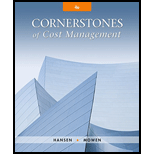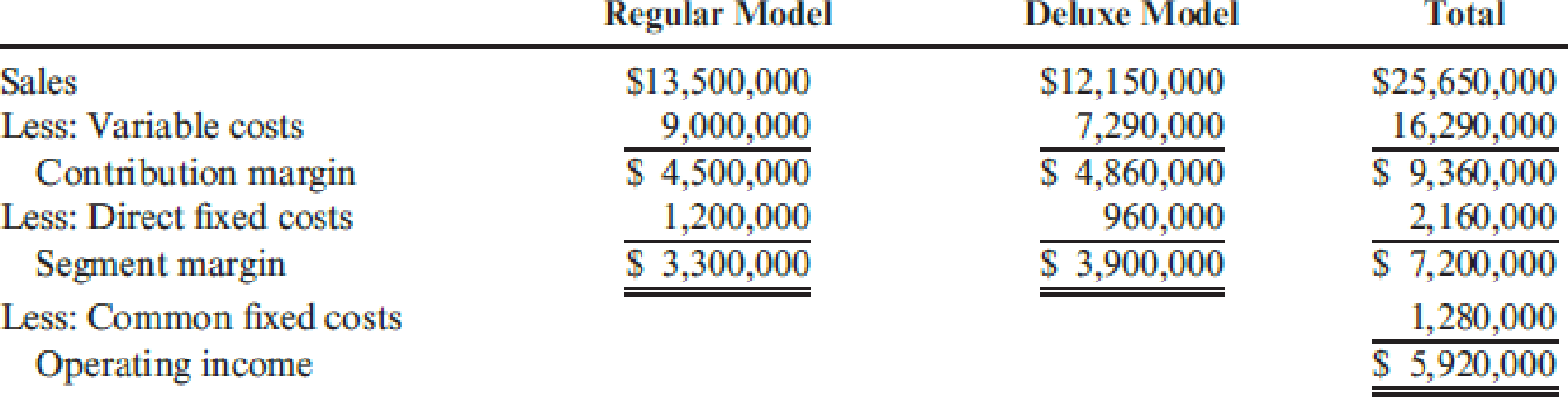# Steinberg Company produces commercial printers. One is the regular model, a basic model that is designed to copy and print in black and white. Another model, the deluxe model, is a color printer-scanner-copier. For the coming year, Steinberg expects to sell 90,000 regular models and 18,000 deluxe models. A segmented income statement for the two products is as follows: Required: 1. Compute the number of regular models and deluxe models that must be sold to break even. 2. Using information only from the total column of the income statement, compute the sales revenue that must be generated for the company to break even. (Round the contribution margin ratio to four significant digits and the sales revenue to the nearest dollar.)### Cornerstones of Cost Management (C...

4th Edition
Don R. Hansen + 1 other
Publisher: Cengage Learning
ISBN: 9781305970663

#### Solutions

Chapter
Section### Cornerstones of Cost Management (C...

4th Edition
Don R. Hansen + 1 other
Publisher: Cengage Learning
ISBN: 9781305970663
Chapter 16, Problem 22E
Textbook Problem
379 views

## Steinberg Company produces commercial printers. One is the regular model, a basic model that is designed to copy and print in black and white. Another model, the deluxe model, is a color printer-scanner-copier. For the coming year, Steinberg expects to sell 90,000 regular models and 18,000 deluxe models. A segmented income statement for the two products is as follows:Required: 1. Compute the number of regular models and deluxe models that must be sold to break even. 2. Using information only from the total column of the income statement, compute the sales revenue that must be generated for the company to break even. (Round the contribution margin ratio to four significant digits and the sales revenue to the nearest dollar.)

1.

To determine

Identify the number of units to be sold for break-even.

### Explanation of Solution

Sales mix: Sales mix refers to relative distribution of the total sales amongst the total number of units sold by a company. It is also expressed as a percentage of units sold for each product produced with respect to the total units sold for all the products produced.

Compute the package contribution margin:

 Input Price (A) Unit Variable cost (B) Contribution margin (C = A−B) Sales Mix (D) Package Contribution margin (E = C × D) Regular Model $150$100 $50 5$250 Deluxe Model $675$405 $270 1$270 Package Total $520 Table (1) Compute the break-even packages: Break-even packages =Total fixed costPackage Contribution margin=$3,440,000\$520=6,615.3846 packages

The number of break-even packages is 6,615.3846.

Compute the break-even regular model:

Break-even regular model =Sales mix ×Break-even packages=5 × 6,615

2.

To determine

Calculate the sales revenue required to generate break-even.

### Still sussing out bartleby?

Check out a sample textbook solution.

See a sample solution

#### The Solution to Your Study Problems

Bartleby provides explanations to thousands of textbook problems written by our experts, many with advanced degrees!

Get Started

Find more solutions based on key concepts
Explain the separate entity concept.

College Accounting (Book Only): A Career Approach

List and describe four determinants of a countrys productivity.

Principles of Economics (MindTap Course List)

What is a systems flowchart?

Accounting Information Systems

Suppose interest rates on Treasury bonds rose from 5% to 9% as a result of higher interest rates in Europe. Wha...

Fundamentals of Financial Management, Concise Edition (with Thomson ONE - Business School Edition, 1 term (6 months) Printed Access Card) (MindTap Course List)